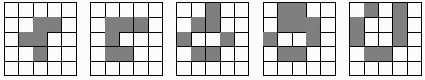# 1103

This number is a prime.Ramanujan produced a curious approximation by dividing 992 by 1103. The quotient being close to 2π times the square root of 2. [Croll]11032 = 1216609 and 30112 = 9066121. [Kulsha]The smallest Sophie Germain prime to be the sum of four consecutive primes. [Post]John Conway's game of life starts with cells that are "alive" (marked or shaded) in an infinite array. Each subsequent "generation" (iteration): a living cell stays alive if it has either two or three living neighbors; and a dead cell springs to life if it has exactly three live neighbors. The R-pentomino and its next four generations are shown in the figure above. The pattern that this pentomino generates does not stabilize until the 1103rd generation. (There is even an initial arrangement of cells that will generate the prime numbers!) [Caldwell]The only four-digit prime (emirp) that is the sum of the reversals of two consecutive primes, i.e., 199 and 211. [Silva]Seymour R. Cray designed the UNIVAC 1103, the first electronically digital computer to become commercially available. He often joked that he was "an overpaid plumber" because most of the computer design revolved around designing cooling units to extract heat from the hardware.(1103, 1217, 1259) is the first case of three consecutive emirps in the sequence of primes that create “apocalyptic numbers”, (2^1103, 2^1217, 2^1259), i.e., containing the beast number in their decimal expansion. [Loungrides]The first 4-digit prime such that the first half of the digits and the second half of the digits are both primes. [Giannopoulos]The smallest prime number that has the same Collatz trajectory length (44) as its prime index (185). [Gaydos]

(There are 3 curios for this number that have not yet been approved by an editor.)